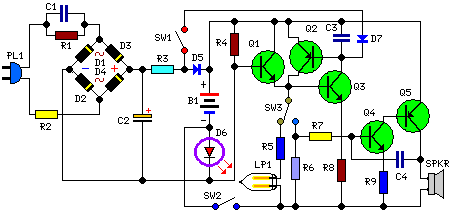# Learning Electronics

Learn to build electronic circuits

# Emergency Light and Alarm

Powered by two AA NI-CD batteries, Four switchable options

This circuit is permanently plugged into a mains socket and NI-CD batteries are trickle-charged. When a power outage occurs, the lamp automatically illuminates. Instead of illuminating a lamp, an alarm sounder can be chosen. When power supply is restored, the lamp or the alarm is switched-off. A switch provides a "latch-up" function, in order to extend lamp or alarm operation even when power is restored.

Circuit Diagram:Parts:

R1 = 220K
R2 = 470R
R3 = 390R
R4 = 1.5K
R5 = 1R
R6 = 10K
R7 = 330K
R8 = 470R
R9 = 100R
D1 = 1N4007
D2 = 1N4007
D3 = 1N4007
D4 = 1N4007
D5 = 1N4007
D6 = Led
D7 = 1N4148
Q1 = BC547
Q2 = BC327
Q3 = BC547
Q4 = BC547
Q5 = BC327
C1 = 330nF-400V
C2 = 10uF-63V
C3 = 100nF-63V
C4 = 10nF-63V
LP1 = 2.5V-300mA Torch Lamp Bulb
PL1 = Male Mains Plug
SW1 = SPST Switches
SW2 = SPST Switches
SW3 = SPDT Switches
SPKR = 8 Ohms Loudspeaker
B1 = 2.5V Battery (two AA NI-CD rechargeable cells wired in series)

Circuit operation:

Mains voltage is reduced to about 12V DC at C2's terminals, by means of the reactance of C1 and the diode bridge (D1-D4). This avoids the use of a mains transformer.
Trickle-charging current for the battery B1 is provided by the series resistor R3, D5 and the green LED D6 that also monitors the presence of mains supply and correct battery charging.
Q2 & Q3 form a self-latching pair that start operating when a power outage occurs. In this case, Q1 biasing becomes positive, so this transistor turns on the self latching pair.
If SW3 is set as shown in the circuit diagram, the lamp illuminates via SW2, which is normally closed; if set the other way, a square wave audio frequency generator formed by Q4, Q5 and related components is activated, driving the loudspeaker.
If SW1 is left open, when mains supply is restored the lamp or the alarm continue to operate. They can be disabled by opening the main on-off switch SW2.
If SW1 is closed, restoration of the mains supply terminates lamp or alarm operation, by applying a positive bias to the Base of Q2.

Notes:
• Close SW2 after the circuit is plugged.
• Warning! The circuit is connected to 230Vac mains, then some parts in the circuit board are subjected to lethal potential!. Avoid touching the circuit when plugged and enclose it in a plastic box.

Source : www.redcircuits.com